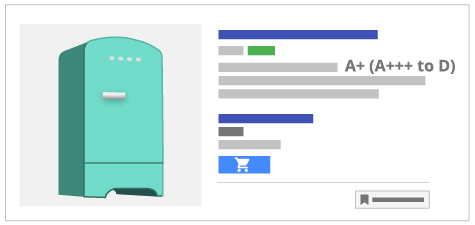# energy_efficiency_class [能效等级]、min_energy_efficiency_class [最低能效等级]、max_energy_efficiency_class [最高能效等级]：定义## 适用情形

`energy_efficiency_class [能效等级]``min_energy_efficiency_class [最低能效等级]``max_energy_efficiency_class [最高能效等级]` 属性是可选属性，但某些地方的法律法规可能会要求您必须提供这些属性。例如，如果您的商品定位至任意欧盟成员国或瑞士，请考虑欧盟指令 2017/1369 的国家实施法案及任何其他适用地方法律对此问题的法律要求。

• 冰箱
• 冰柜
• 洗衣机
• 滚筒干衣机
• 洗衣干衣一体机
• 葡萄酒存储设备
• 洗碗机
• 烤箱
• 热水器
• 热水存储设备
• 空调
• 电灯泡
• 灯具
• 电视

## 格式

• A+++
• A++
• A+
• A
• B
• C
• D
• E
• F
• G

#### energy_efficiency_class [能效等级] 格式

`A+`

XML Feed ```  <g:energy_efficiency_class>A+</g:energy_efficiency_class>```

#### min_energy_efficiency_class [最低能效等级] 格式

A+

XML Feed ```  <g:min_energy_efficiency_class>A</g:min_energy_efficiency_class>```

#### max_energy_efficiency_class [最高能效等级] 格式

`D`

XML Feed ```  <g:max_energy_efficiency_class>D</g:max_energy_efficiency_class>```

## 指南

### 最低要求

• `min_energy_efficiency_class [最低能效等级]` 值必须小于 `max_energy_efficiency_class [最高能效等级]`。例如，您的能效等级一般会按照从 A 到 D（而非从 D 到 A）排列。
• 您提供的 `energy_efficiency_class [能效等级]` 值必须介于 `min_efficiency_class [最低能效等级]` 值与 `max_energy_efficiency_class [最高能效等级]` 值之间。例如，如果您的能效等级为 A 到 D，则您的 `energy_efficiency_class [能效等级]` 值不能是 G。

### 最佳做法

• 提交 `unit_pricing_measure [价格计量单位]``energy_efficiency_class [能效等级]` 属性；二者不能同时提交。如果两个属性都有，则将只显示 `energy_efficiency_class [能效等级]`

## 示例

 商品 不锈钢冰箱 `title [商品名]` 不锈钢冰箱 - 240 升 `energy_efficiency_class [能效等级]` A+ `min_energy_efficiency_class [最低能效等级]` A+++ `max_energy_efficiency_class [最高能效等级]` D

 商品 洗碗机 `title [商品名]` 内置洗碗机 - 宽：60 cm，深：55 cm `energy_efficiency_class [能效等级]` A++ `min_energy_efficiency_class [最低能效等级]` A+++ `max_energy_efficiency_class [最高能效等级]` G

 商品 酒柜 `title [商品名]` 酒柜 - 宽：45 cm，深：52 cm `energy_efficiency_class [能效等级]` B `min_energy_efficiency_class [最低能效等级]` A `max_energy_efficiency_class [最高能效等级]` F